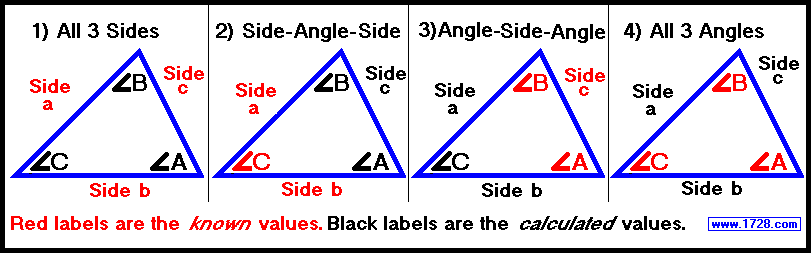Ultimate Triangle
CalculatorAngles are in or
Then click on the triangle information to be input.
>>>>>
>>>>>
>>>>>

The graphic at the top of this page depicts 4 instances of solving for ANY type of triangle.
 Diagram 1 is self-explanatory. Diagram 2 (abbreviated S-A-S) You must use an included angle (the angle between the two sides). Diagram 3 (abbreviated A-S-A) You must use an included side (the side between the two angles). Diagram 4 Only the ratio of the 3 sides can be determined. If you input the smallest angle in the "ANGLE 1" box, then the shortest side will have a value of 1. If you input the largest angle in the "ANGLE 1" box, then the longest side will have a value of 1. Note: If you are given 3 angles and they sum to 180° they will always form a triangle.

 To clear the boxes to input more data, click any of the 4 numbered "choice" buttons. Here's a neat time-saver for you. Click button number 1, input 3 sides, then click calculate. The default is degrees but let's suppose you wanted radians. Click on 'radians', do NOT click on button 1 again but click on 'calculate'. Pretty neat huh? If you did this with choice number 2, you would have to change one input box to radians, and with choice 3, you would have to change two input boxes to radians. Significant Figures >>> Numbers are displayed in scientific notation with the amount of significant figures you specify. For easier readability, numbers between .001 and 1,000 will not be in scientific notation but will still have the same precision. You may change the number of significant figures displayed by changing the number in the box above. Most browsers, will display the answers properly but if you are seeing no answers at all, enter a zero in the box above, which will eliminate all formatting but at least you will see the answers.